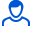Content: teorver_5571.doc (28.00 KB)

Positive responses: 0
Negative responses: 0

Refunds: 05571. x̅=3, n=400, σ=29. Determine the confidence interval for estimating with reliability γ=0.94 the unknown mathematical expectation a of the normally distributed feature X of the general population, if the sample mean x̅в=3, the sample size n=400 and the general standard deviation σ=29 are known.
Detailed solution. Decorated in Microsoft Word 2003 (Quest decided to use the formula editor)
No feedback yet